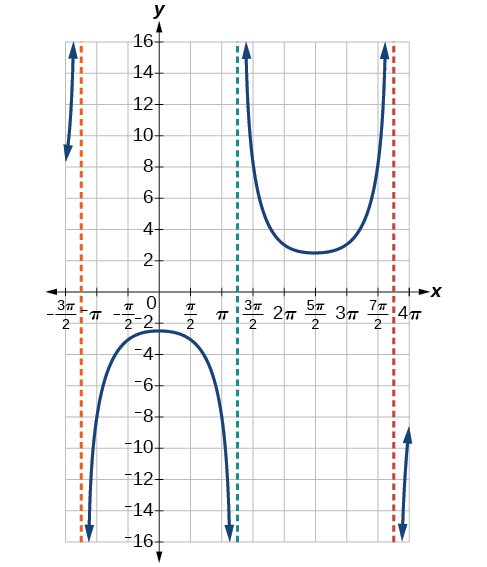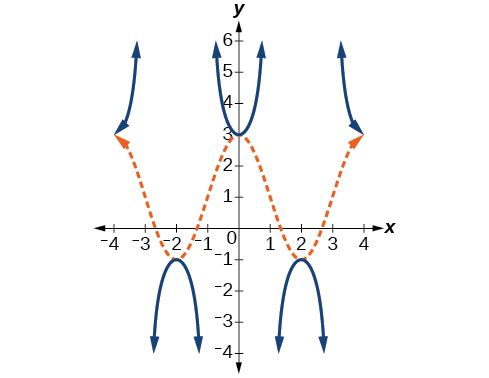# 8.2 Graphs of the other trigonometric functions  (Page 6/9)

 Page 6 / 9

The domain of $\text{\hspace{0.17em}}\mathrm{csc}\text{\hspace{0.17em}}x\text{\hspace{0.17em}}$ was given to be all $\text{\hspace{0.17em}}x\text{\hspace{0.17em}}$ such that $\text{\hspace{0.17em}}x\ne k\pi \text{\hspace{0.17em}}$ for any integer $\text{\hspace{0.17em}}k.\text{\hspace{0.17em}}$ Would the domain of $\text{\hspace{0.17em}}y=A\mathrm{csc}\left(Bx-C\right)+D\text{\hspace{0.17em}}\text{be}\text{\hspace{0.17em}}x\ne \frac{C+k\pi }{B}?$

Yes. The excluded points of the domain follow the vertical asymptotes. Their locations show the horizontal shift and compression or expansion implied by the transformation to the original function’s input.

Given a function of the form $\text{\hspace{0.17em}}y=A\mathrm{csc}\left(Bx\right),\text{\hspace{0.17em}}$ graph one period.

1. Express the function given in the form $\text{\hspace{0.17em}}y=A\mathrm{csc}\left(Bx\right).$
2. $\text{\hspace{0.17em}}|A|.$
3. Identify $\text{\hspace{0.17em}}B\text{\hspace{0.17em}}$ and determine the period, $\text{\hspace{0.17em}}P=\frac{2\pi }{|B|}.$
4. Draw the graph of $\text{\hspace{0.17em}}y=A\mathrm{sin}\left(Bx\right).$
5. Use the reciprocal relationship between $\text{\hspace{0.17em}}y=\mathrm{sin}\text{\hspace{0.17em}}x\text{\hspace{0.17em}}$ and $\text{\hspace{0.17em}}y=\mathrm{csc}\text{\hspace{0.17em}}x\text{\hspace{0.17em}}$ to draw the graph of $\text{\hspace{0.17em}}y=A\mathrm{csc}\left(Bx\right).$
6. Sketch the asymptotes.
7. Plot any two reference points and draw the graph through these points.

## Graphing a variation of the cosecant function

Graph one period of $\text{\hspace{0.17em}}f\left(x\right)=-3\mathrm{csc}\left(4x\right).$

• Step 1. The given function is already written in the general form, $\text{\hspace{0.17em}}y=A\mathrm{csc}\left(Bx\right).$
• Step 2. $\text{\hspace{0.17em}}|A|=|-3|=3,$ so the stretching factor is 3.
• Step 3. $\text{\hspace{0.17em}}B=4,$ so $\text{\hspace{0.17em}}P=\frac{2\pi }{4}=\frac{\pi }{2}.\text{\hspace{0.17em}}$ The period is $\text{\hspace{0.17em}}\frac{\pi }{2}\text{\hspace{0.17em}}$ units.
• Step 4. Sketch the graph of the function $\text{\hspace{0.17em}}g\left(x\right)=-3\mathrm{sin}\left(4x\right).$
• Step 5. Use the reciprocal relationship of the sine and cosecant functions to draw the cosecant function .
• Steps 6–7. Sketch three asymptotes at $\text{\hspace{0.17em}}x=0,\text{\hspace{0.17em}}x=\frac{\pi }{4},\text{\hspace{0.17em}}$ and $\text{\hspace{0.17em}}x=\frac{\pi }{2}.\text{\hspace{0.17em}}$ We can use two reference points, the local maximum at $\text{\hspace{0.17em}}\left(\frac{\pi }{8},-3\right)\text{\hspace{0.17em}}$ and the local minimum at $\text{\hspace{0.17em}}\left(\frac{3\pi }{8},3\right).$ [link] shows the graph.

Graph one period of $\text{\hspace{0.17em}}f\left(x\right)=0.5\mathrm{csc}\left(2x\right).$Given a function of the form $\text{\hspace{0.17em}}f\left(x\right)=A\mathrm{csc}\left(Bx-C\right)+D,\text{\hspace{0.17em}}$ graph one period.

1. Express the function given in the form $\text{\hspace{0.17em}}y=A\mathrm{csc}\left(Bx-C\right)+D.$
2. Identify the stretching/compressing factor, $\text{\hspace{0.17em}}|A|.$
3. Identify $\text{\hspace{0.17em}}B\text{\hspace{0.17em}}$ and determine the period, $\text{\hspace{0.17em}}\frac{2\pi }{|B|}.$
4. Identify $\text{\hspace{0.17em}}C\text{\hspace{0.17em}}$ and determine the phase shift, $\text{\hspace{0.17em}}\frac{C}{B}.$
5. Draw the graph of $\text{\hspace{0.17em}}y=A\mathrm{csc}\left(Bx\right)\text{\hspace{0.17em}}$ but shift it to the right by and up by $\text{\hspace{0.17em}}D.$
6. Sketch the vertical asymptotes, which occur at $\text{\hspace{0.17em}}x=\frac{C}{B}+\frac{\pi }{|B|}k,$ where $\text{\hspace{0.17em}}k\text{\hspace{0.17em}}$ is an integer.

## Graphing a vertically stretched, horizontally compressed, and vertically shifted cosecant

Sketch a graph of $\text{\hspace{0.17em}}y=2\mathrm{csc}\left(\frac{\pi }{2}x\right)+1.\text{\hspace{0.17em}}$ What are the domain and range of this function?

• Step 1. Express the function given in the form $\text{\hspace{0.17em}}y=2\mathrm{csc}\left(\frac{\pi }{2}x\right)+1.$
• Step 2. Identify the stretching/compressing factor, $\text{\hspace{0.17em}}|A|=2.$
• Step 3. The period is $\text{\hspace{0.17em}}\frac{2\pi }{|B|}=\frac{2\pi }{\frac{\pi }{2}}=\frac{2\pi }{1}\cdot \frac{2}{\pi }=4.$
• Step 4. The phase shift is $\text{\hspace{0.17em}}\frac{0}{\frac{\pi }{2}}=0.$
• Step 5. Draw the graph of $\text{\hspace{0.17em}}y=A\mathrm{csc}\left(Bx\right)\text{\hspace{0.17em}}$ but shift it up $\text{\hspace{0.17em}}D=1.$
• Step 6. Sketch the vertical asymptotes, which occur at $\text{\hspace{0.17em}}x=0,x=2,x=4.$

The graph for this function is shown in [link] .

Given the graph of $\text{\hspace{0.17em}}f\left(x\right)=2\mathrm{cos}\left(\frac{\pi }{2}x\right)+1\text{\hspace{0.17em}}$ shown in [link] , sketch the graph of $\text{\hspace{0.17em}}g\left(x\right)=2\mathrm{sec}\left(\frac{\pi }{2}x\right)+1\text{\hspace{0.17em}}$ on the same axes.## Analyzing the graph of y = cot x

The last trigonometric function we need to explore is cotangent    . The cotangent is defined by the reciprocal identity $\text{\hspace{0.17em}}\mathrm{cot}\text{\hspace{0.17em}}x=\frac{1}{\mathrm{tan}\text{\hspace{0.17em}}x}.\text{\hspace{0.17em}}$ Notice that the function is undefined when the tangent function is 0, leading to a vertical asymptote in the graph at $\text{\hspace{0.17em}}0,\pi ,\text{\hspace{0.17em}}$ etc. Since the output of the tangent function is all real numbers, the output of the cotangent function is also all real numbers.

We can graph $\text{\hspace{0.17em}}y=\mathrm{cot}\text{\hspace{0.17em}}x\text{\hspace{0.17em}}$ by observing the graph of the tangent function because these two functions are reciprocals of one another. See [link] . Where the graph of the tangent function decreases, the graph of the cotangent function increases. Where the graph of the tangent function increases, the graph of the cotangent function decreases.

#### Questions & Answers

write down the polynomial function with root 1/3,2,-3 with solution
if A and B are subspaces of V prove that (A+B)/B=A/(A-B)
write down the value of each of the following in surd form a)cos(-65°) b)sin(-180°)c)tan(225°)d)tan(135°)
Prove that (sinA/1-cosA - 1-cosA/sinA) (cosA/1-sinA - 1-sinA/cosA) = 4
what is the answer to dividing negative index
In a triangle ABC prove that. (b+c)cosA+(c+a)cosB+(a+b)cisC=a+b+c.
give me the waec 2019 questions
the polar co-ordinate of the point (-1, -1)
prove the identites sin x ( 1+ tan x )+ cos x ( 1+ cot x )= sec x + cosec x
tanh`(x-iy) =A+iB, find A and B
B=Ai-itan(hx-hiy)
Rukmini
what is the addition of 101011 with 101010
If those numbers are binary, it's 1010101. If they are base 10, it's 202021.
Jack
extra power 4 minus 5 x cube + 7 x square minus 5 x + 1 equal to zero
the gradient function of a curve is 2x+4 and the curve passes through point (1,4) find the equation of the curve
1+cos²A/cos²A=2cosec²A-1
test for convergence the series 1+x/2+2!/9x3ByByByBy Mldelatte